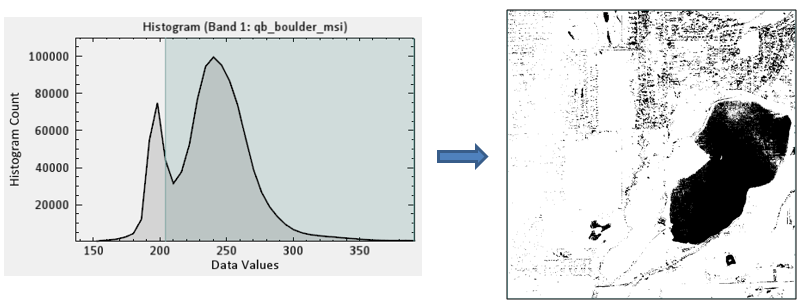This task creates a new raster where values above a specified threshold are set to 1 and all other values are set to 0. The task uses a predefined thresholding method to create the binary image. Thresholds are calculated for each band in the source raster. Image thresholding is typically done to separate "object" or foreground pixels from background pixels to aid in image processing.## Example

`; Start the application`
`e = ENVI()`
` `
`; Open an input file`
`File = Filepath('qb_boulder_msi', Subdir=['data'], \$`
`  Root_Dir=e.Root_Dir)`
`Raster = e.OpenRaster(File)`
` `
`; Get the task from the catalog of ENVITasks`
`Task = ENVITask('BinaryAutomaticThresholdRaster')`
` `
`; Define inputs`
`Task.INPUT_RASTER = Raster`
`Task.METHOD = 'Minimum Error'`
` `
`; Run the task`
`Task.Execute`
` `
`; Add the output to the Data Manager`
`e.Data.Add, Task.OUTPUT_RASTER`
` `
`; Display the result`
`View = e.GetView()`
`Layer = View.CreateLayer(Task.OUTPUT_RASTER)`

## Syntax

Input properties (Set, Get): INPUT_RASTER, INVERSE, METHOD, OUTPUT_RASTER_URI

Output properties (Get only): OUTPUT_RASTER

Properties marked as "Set" are those that you can set to specific values. You can also retrieve their current values any time. Properties marked as "Get" are those whose values you can retrieve but not set.

## Properties

This task also contains the following properties:

### INPUT_RASTER (required)

Specify the raster to threshold.

### INVERSE (optional)

The default value for this property is false, which means that values above the computed threshold are set to 1 and all other values are set to 0. Set this property to true to invert the binary values.

### METHOD (optional)

Specify one of the following strings indicating the method used to calculate the threshold.

• Isodata: This method works iteratively by calculating an initial threshold that is half the dynamic range of the image or layer, effectively dividing the image into "foreground" (above the initial threshold) and "background" (below the initial threshold) pixels. Next, the algorithm separately calculates the sample mean of the foreground and background pixels, using these new sample means to calculate a new threshold value (the average of the sample means). The process repeats using each new, successive threshold value until the resulting threshold value ceases to change (Ridler and Calvard, 1978).
• Mean: This method takes the mean value of the gray levels as the threshold (Glasbey, 1993).
• Maximum Entropy: This method considers the thresholding image as two classes of events, with each class characterized by a Probability Density Function (PDF). It then maximizes the sum of the entropy of the two PDFs to converge on a single threshold value (Kapur, Sahoo, and Wong, 1985).
• Minimum Error: This method approximates the histogram as a bimodal Gaussian distribution and finds a cutoff point. The cost function is based on the Bayes classification rule (Kittler and Illingworth, 1986).
• Moments: This method considers the grayscale image as a blurred version of an ideal binary image. This method determines the threshold so that the first three moments of the input image are preserved in the output image (Tsai, 1985).
• Otsu (default): A histogram shape-based method. It is based on discriminate analysis and uses the zero- and the first-order cumulative moments of the histogram for calculating the value of the thresholding level (Otsu, 1979).

### OUTPUT_RASTER

This is a reference to the output raster of filetype ENVI.

### OUTPUT_RASTER_URI (optional)

Specify a string with the fully qualified filename and path to export the associated OUTPUT_RASTER.

• If you set this property to an asterisk symbol (*), the output raster will be virtual and not written to disk.
• If you do not specify this property, or set it to an exclamation symbol (!), a temporary file will be created.

## References

Glasbey, C. "An Analysis of Histogram-Based Thresholding Algorithms." CVGIP: Graphical Models and Image Processing 55 (1993): 532-537.

Kapur, J., P. Sahoo, and A. Wong. "A New Method for Gray-Level Picture Thresholding Using the Entropy of the Histogram." Graphical Models and Image Processing 29, No. 3 (1985): 273-285.

Kittler, J., and J. Illingworth. "Minimum Error Thresholding." Pattern Recognition 19 (1986): 41-47.

Otsu, N. "A Threshold Selection Method from Gray-Level Histograms." IEEE Transactions on Systems, Man and Cybernetics 9 (1979): 62–66.

Ridler, T., and S. Calvard. "Picture Thresholding Using an Iterative Selection Method." IEEE Transactions on Systems, Man and Cybernetics 8 (1978): 630 - 632.

Tsai, W. "Moment-Preserving Thresholding: a New Approach." Computer Vision, Graphics, and Image Processing 29 (1985): 377-393.

## Version History

 ENVI 5.5. 2 Introduced

4.2